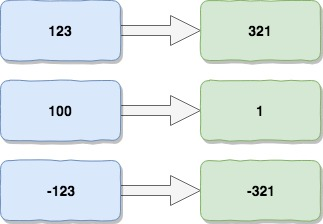# 整数反转 Reverse Integer

## 题目

Given a 32-bit signed integer, reverse digits of an integer.

Example 1:

``````Input: 123
Output: 321
``````

Example 2:

``````Input: -123
Output: -321
``````

Example 3:

``````Input: 120
Output: 21
``````

Note:

Assume we are dealing with an environment which could only store integers within the 32-bit signed integer range: [−2^31, 2^31 − 1]. For the purpose of this problem, assume that your function returns 0 when the reversed integer overflows.

## 思路&题解``````public class Solution {
public int reverse(int x) {
int min = -1<<31;
int max = -min - 1;
int result = 0;
while(x != 0) {
// 取余数，相当于获取低位数值
int rightPart = x%10;
// 易错点：除以10，相当于移动最低位,
x /= 10;
// 易错点：此处可能会溢出
//result = result * 10 + rightPart;
if (result > max/10 || (result == max/10 && rightPart > 7)) {
return 0;
}
if (result < min/10 || (result == min/10 && rightPart < -8)) {
return 0;
}
result = result * 10 + rightPart;
}
return result;
}
}
``````

## 知识点分析

• 逐位处理

`tmp = x%10`

• 位增减处理

`x /= 10`

• 整型溢出原理

``````// 易错点：此处可能会溢出
result = result * 10 + rightPart;
``````
1. 如果上述语句溢出，由于rightPart是取值为[0,9],不可能溢出，因此溢出的一定是`result * 10`
2. 如果`result * 10`溢出，则`result * 10 >= max` 或者 `result * 10 =< min`，等于不一定会溢出，需要结合rightPart的取值；

1. 如果`result * 10 >= max`，则`result >= max/10`
2. `result > max/10`时，一定是溢出的
3. `result == max/10`时，需要结合rightPart，即两者只和如果大于max就溢出
4. 因为max的个位数是7，所以`rightPart > 7`时溢出
5. 整合起来就是第一个if的逻辑块

1. 如果`result * 10 <= min`，则`result <= min/10`
2. `result < min/10`时，一定是溢出的
3. `result == min/10`时，需要结合rightPart，即两者只和如果小于min就溢出
4. 因为min的个位数是8，所以`rightPart < -8`时溢出
5. 整合起来就是第二个if的逻辑块

``````System.out.println("max = " + max + "，" + Integer.toBinaryString(max) + ", "+ max%10);
System.out.println("min = " + min + "，" + Integer.toBinaryString(min) +"，"+min%10);
``````

``````max = 2147483647，1111111111111111111111111111111, 7
min = -2147483648，10000000000000000000000000000000，-8
``````